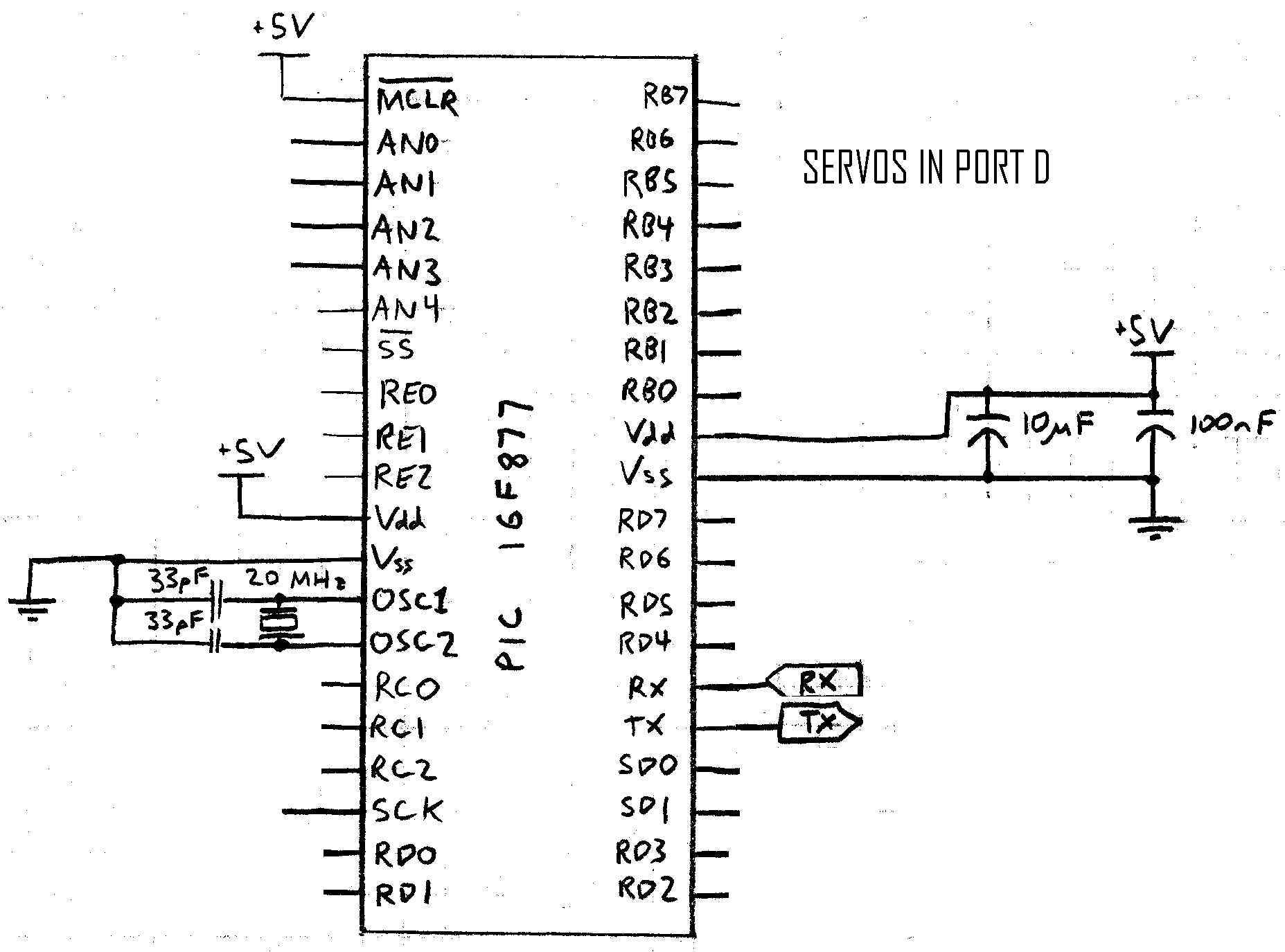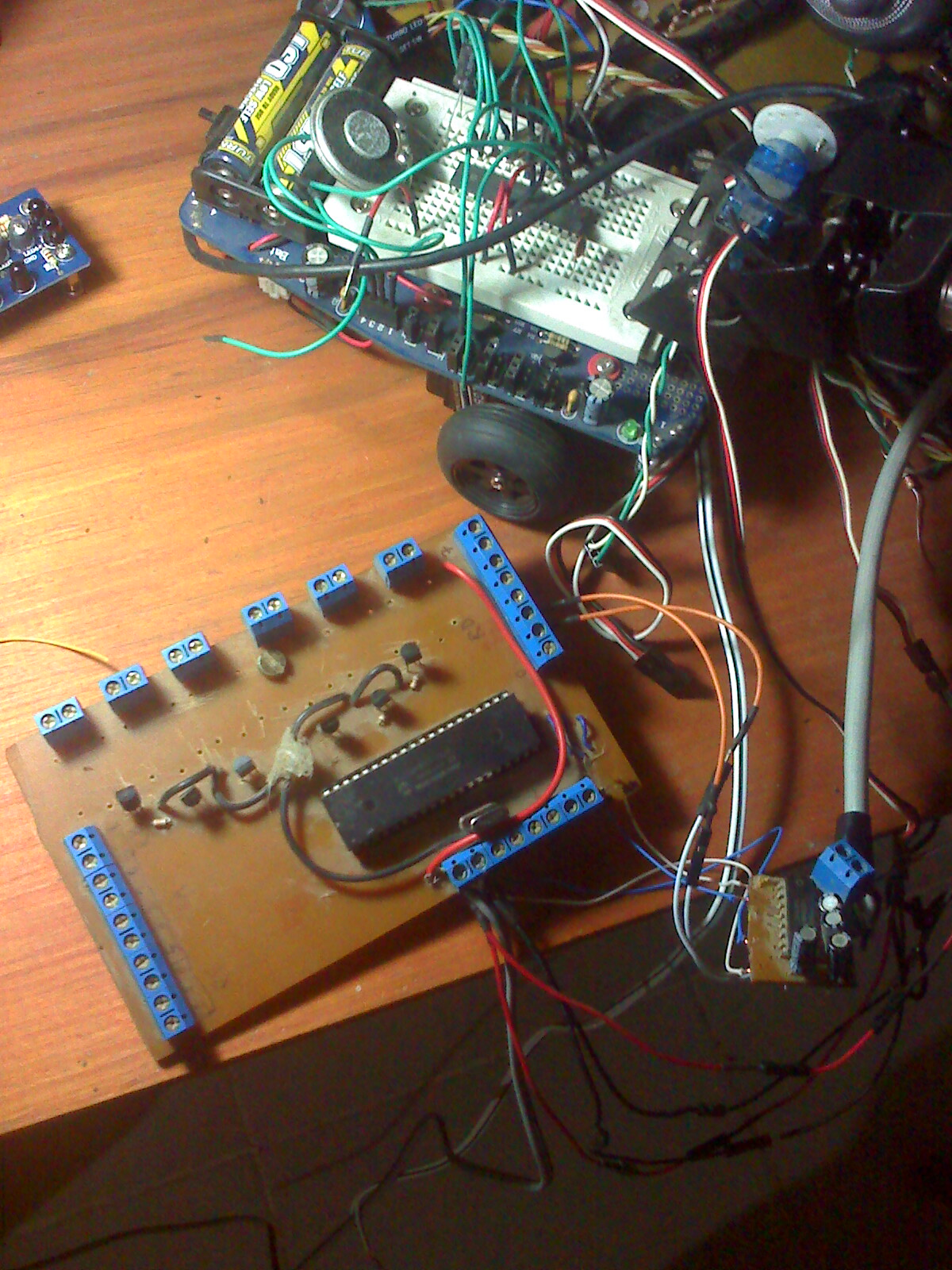# I need to read the serial port and print it Phithon

hello brothers, I need to read the serial port and print it Phithon , but did not find examples of how to read .

want to drive a servo controller that I made ​​with PIC via serial port.

write with google translate hope understand.### Hello mecax, you could take a

Hello mecax,

you could take a look at the Serial service -> http://myrobotlab.org/service/serial

some examples:

but we can help you better, if you share a few more details with us.

I don't know about you servo controller, maybe we could "borg" it in.

Text, Links, Pictures, (Videos) - all that helps a lot!### aaa

hello ma. vo., If I think it's the example serial.ascitochar which receives the signal from the port so that you can discuss with Python. and proves it and I'll upload them to the project I'm doing.

Thanks.### Great, that it helped,

Great, that it helped, although I just realized, that there is prossibly an error in it.

If so, try this:

```#file : Serial.asciiToChar.py
import time

#create a Serial service named serial
serial = Runtime.createAndStart("serial","Serial")
def input(code):
decoded = ''.join(chr(code))
print decoded
#have python listening to serial
#connect to a serial port COM4 57600 bitrate 8 data bits 1 stop bit 0 parity
serial.connect("COM9", 57600, 8, 1, 0)
#sometimes its important to wait a little for hardware to get ready
sleep(1)

#write a series of bytes to the serial port
serial.write(87)
serial.write(79)
serial.write(82)
serial.write(75)
serial.write(89)
serial.write(32)```

Maybe you want to share details of the project you are doing? Here are a lot of people on MRL, that have many ideas and could improve it. Also it is very interesting to see, what others are doing and get ideas form it/them.### THIS IS THE PROJECT

I tell them what the idea of ​​my proyecto.Yo have done a controlling 16 servos with the pic I sent him 2 decimal data serial port, the first thing is a number that defines the pin q is a servo to and the second control data is a number of 5 to 25 which is the equivalent of 0.5 ms and 2.5 ms of the pulses with the servos are handled. In this controller are connected bread and servos tilt webcam and want you to continue face the same manner as they apply with arduino. Already achieved with the controlling handle MRL is providing me help me put the data between services opencv and serial.

THIS IS A PICTURE OF THE PIC:and this is recorded on the pic in PICC language:

#include <16f877.h>
#fuses HS,NOWDT,NOPROTECT,NOLVP,NOBROWNOUT,PUT
#use delay (clock=20000000)
#USE RS232 (baud=57600,bits=8,parity=N,stop=1,xmit=PIN_C6,rcv=PIN_C7)

#use fast_io(A)
#use fast_io(B)
#use fast_io(C)
#use fast_io(D)
#use fast_io(E)

#byte PORTA      = 0x05
#byte PORTB      = 0x06
#byte PORTC      = 0x07
#byte PORTD      = 0x08
#byte PORTE      = 0x09

#bit servo_00     = PORTD.0
#bit servo_01     = PORTD.1
#bit servo_02     = PORTD.2
#bit servo_03     = PORTD.3
#bit servo_04     = PORTD.4
#bit servo_05     = PORTD.5
#bit servo_06     = PORTD.6
#bit servo_07     = PORTD.7

#define n 16// NUMBER OF SERVOS
//for another number of servos to do time measurements

#define max_theCount 200// 200*100us=20ms
#define derecha  25//  25*100us=2,5ms
// It would be a matter of testing with real servos and adjust values
#define centro   15// 15*100us=1,5ms
#define izquierda 5// 5*100us =0,5ms

#define val_timer0 88

int8    theCount=1;
int8    duty[n];
int8    servo_08;
int8    servo_09;
int8    servo_10;
int8    servo_11;
int8    servo_12;
int8    servo_13;
int8    servo_14;
int8    servo_15;

#INT_TIMER0
void isr_timer0(){// Each comes here 100us cyclically
#asm
nop
#endasm
if (duty >= theCount)
{
servo_00=1;
}
else
{
servo_00=0;
#asm
nop
#endasm
}

if (duty >= theCount)
{
servo_01=1;
}
else
{
servo_01=0;
#asm
nop
#endasm
}

if (duty >= theCount)
{
servo_02=1;
}
else
{
servo_02=0;
#asm
nop
#endasm
}

if (duty >= theCount)
{
servo_03=1;
}
else
{
servo_03=0;
#asm
nop
#endasm
}

if (duty >= theCount)
{
servo_04=1;
}
else
{
servo_04=0;
#asm
nop
#endasm
}

if (duty >= theCount)
{
servo_05=1;
}
else
{
servo_05=0;
#asm
nop
#endasm
}

if (duty >= theCount)
{
servo_06=1;
}
else
{
servo_06=0;
#asm
nop
#endasm
}

if (duty >= theCount)
{
servo_07=1;
}
else
{
servo_07=0;
#asm
nop
#endasm
}

if (duty >= theCount)
{
servo_08=1;
}
else
{
servo_08=0;
#asm
nop
#endasm
}

if (duty >= theCount)
{
servo_09=1;
}
else
{
servo_09=0;
#asm
nop
#endasm
}

if (duty >= theCount)
{
servo_10=1;
}
else
{
servo_10=0;
#asm
nop
#endasm
}

if (duty >= theCount)
{
servo_11=1;
}
else
{
servo_11=0;
#asm
nop
#endasm
}

if (duty >= theCount)
{
servo_12=1;
}
else
{
servo_12=0;
#asm
nop
#endasm
}

if (duty >= theCount)
{
servo_13=1;
}
else
{
servo_13=0;
#asm
nop
#endasm
}

if (duty >= theCount)
{
servo_14=1;
}
else
{
servo_14=0;
#asm
nop
#endasm
}

if (duty >= theCount)
{
servo_15=1;
}
else
{
servo_15=0;
#asm
nop
#endasm
}

theCount=theCount+1;
if (theCount>max_theCount)
{
theCount=1;
}
else
{
delay_us(1);
#asm
nop
#endasm
}
set_timer0(val_timer0);
}

/////////////////////////////////////// HOME /////////////////////////////////////

void main()
{
int     k;
int8    i=0;// s the exclusive duty rate [] in the serial reception
int1    band=0;

set_tris_b(0x00);
set_tris_c(0b10000001);
set_tris_d(0x00);
set_tris_e(0x01);

PORTB=0x00;
PORTD=0x00;

port_b_pullups(FALSE);

for(k=0;k<=(n-1);k++)
duty[k]=centro;//

setup_timer_1(T1_DISABLED);
setup_timer_2(T2_DISABLED,0,1);
setup_timer_0(RTCC_DIV_2);

set_timer0(val_timer0);

delay_ms(700);

enable_interrupts(INT_TIMER0);
enable_interrupts(GLOBAL);

while (1)
{
// Recepción de datos
if (kbhit()){
if (band==0){
i=getc();                            //FIRST DATA RECEIVED THAT DEFINE THE CONTROL SERVO
band=1;
}
else{
duty[i]=getc();                      //DEFINE DATA RECEIVED THAT SECOND POSITION SERVO
band=0;
}
}
}
}

and this is the mess of things I have in the shop, controller and servos with pan and tilt webcam is:well I hope to advance the project, I greet you all### Great! Haven't thought, you

Great!

Haven't thought, you were that far, nice project! Keep it going!